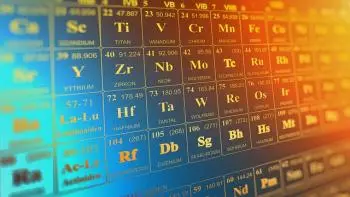# What Is the Atomic Mass?

The atomic mass of a chemical element is the weighted average atomic mass of all isotopes of all single atoms that exist in the nature of an element. It includes protons, neutrons, and electrons. This calculation takes into account its natural prevalence (percentage) in the earth's crust and the atmosphere.It is one of the fundamental properties of a chemical element. The SI unit of measurement is the kilogram (kg). We usually express the mass in grams, although we can also use the unified atomic mass unit (AMU), another unit outside the system. AMU is also called dalton (Da) after John Dalton due to his work in Dalton's atomic model.

This mass is the one presented in D. Mendeleev's periodic table and is used in stoichiometric calculations.

The atomic mass with an altered isotope ratio (for example, enriched in some isotopes) differs from the standard. For monoisotopic elements (such as iodine, gold, etc.), the atomic mass is the atomic mass of its only isotope present in a natural mixture.

For chemical elements absent in nature, the atomic mass of an element indicates the mass number of the most stable isotopes known from this element. They are not in nature because they are synthetic chemical elements. The periodic table traditionally indicates these values in square brackets.

## Excess Mass of an Atom

Not all atoms of an element have the same mass since the different isotopes of each one have a different atomic weight. Therefore, they do not have the same mass number, the sum of the masses of protons and neutrons. In addition to the mass of the difference in the number of protons and neutrons, the mass depends on the binding energy of these sub-particles.

The difference between the isotope's mass and its mass number is called the excess mass (usually expressed in M ​​eV). This value can be both positive and negative.

The dependence of the atomic mass of the isotope on the mass number is as follows: the excess mass is positive for hydrogen-1. With an increase in the mass, the number decreases and becomes negative until it reaches a minimum for iron- 56. Then, it begins to grow and increases to positive values ​​for heavy nuclides.

It corresponds to the fact that fission of nuclei heavier than iron releases energy, while fission of light nuclei requires energy. On the contrary, the fusion of lighter nuclei than iron releases energy, while the fusion of heavier elements than iron requires additional energy.

Do not confuse the mass number with the atomic number. The mass number is the number of neutrons and protons. On the other hand, the atomic number is only the number of protons in a nucleus.

## Relative Atomic Mass

The relative mass shows how many times the mass of a given element is greater than the atomic mass of another element taken as a unit of mass.

Its use is because the absolute mass is a minimal quantity and difficult to handle. Therefore, in chemistry, it is much more convenient to use this relative value.

This value is determined using a carbon atom. The relative mass is defined as the ratio of the mass of the element to 1/12 the mass of the isotope of the carbon-12 atom with a neutral electric charge.

## Mass Spectrometer

Mass spectrometry (MS) is an analytical technique that is used to measure the mass-to-charge ratio of ions. The results are typically presented as a mass spectrum, a plot of intensity as a function of the mass-to-charge ratio.

## Molecular Mass

Molecular mass of a chemical compound is the sum of the atomic masses of the elements that compose it, multiplied by the stoichiometric coefficients of the elements by the compound's chemical formula.

Author:

Published: December 2, 2019
Last review: May 26, 2023